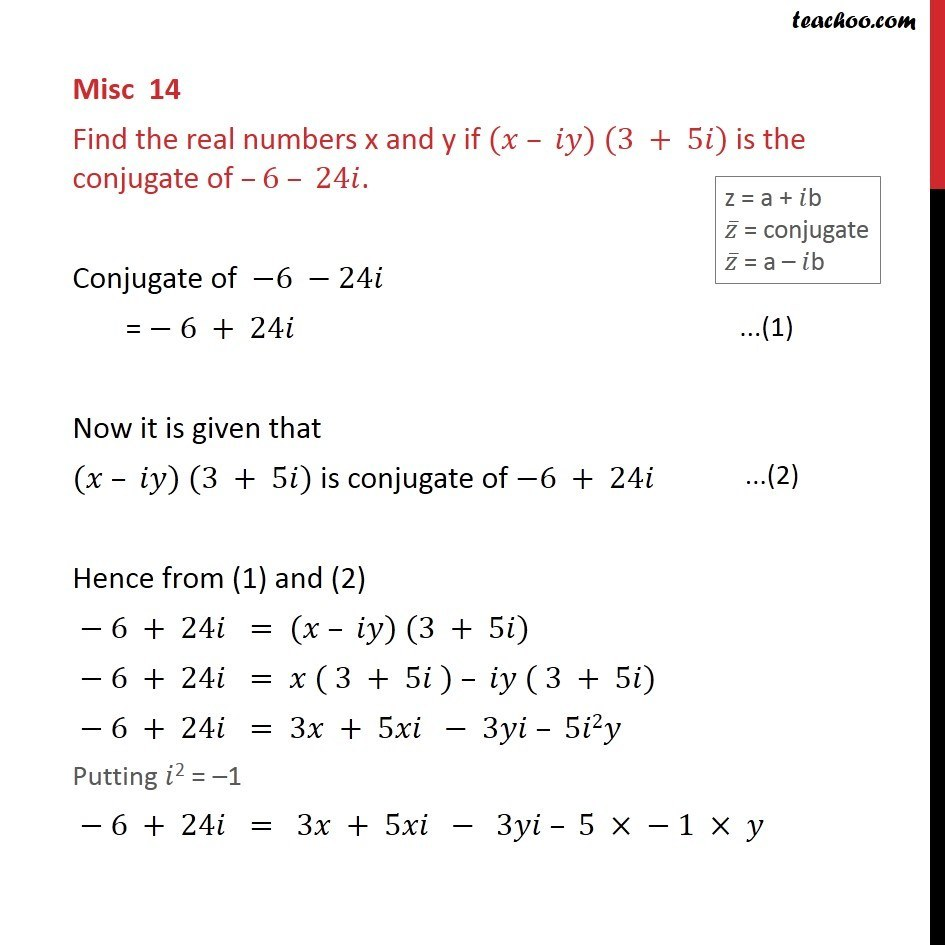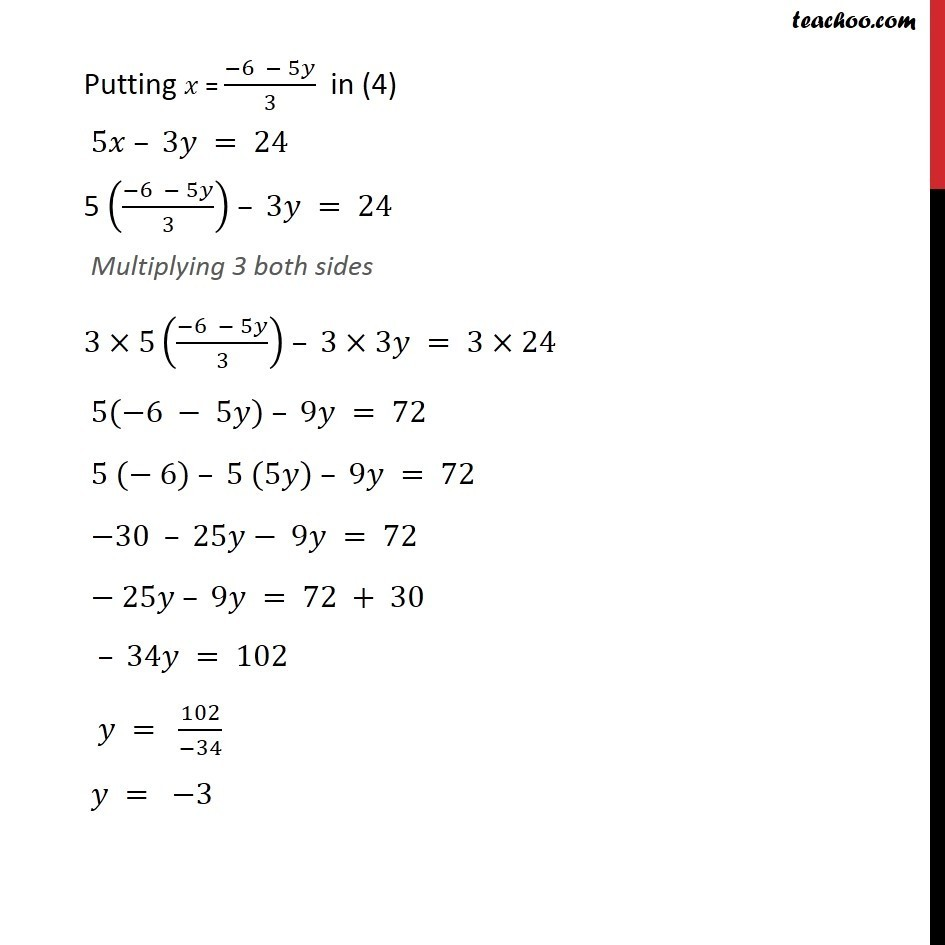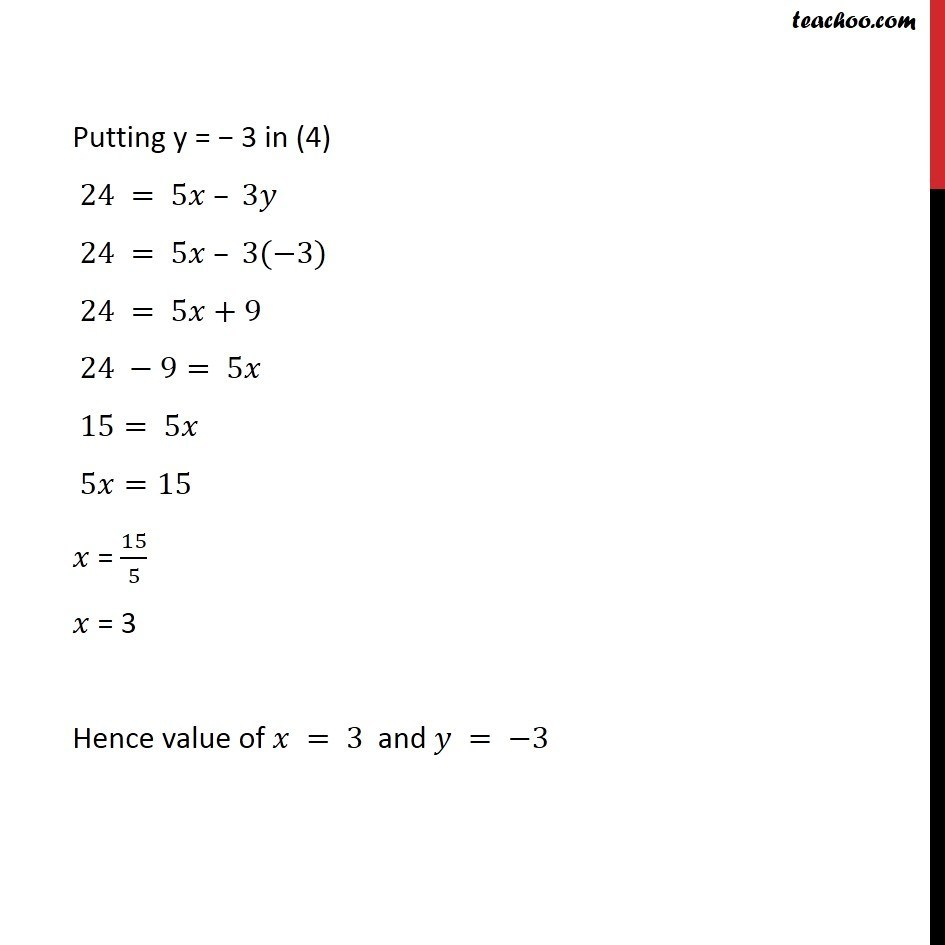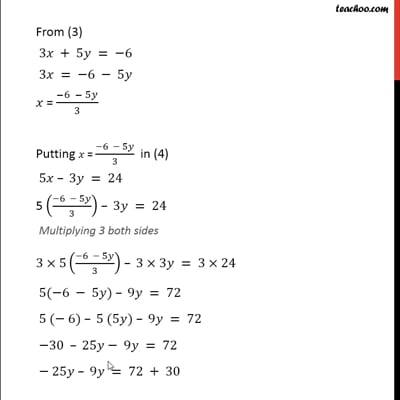Miscellaneous

Chapter 5 Class 11 Complex Numbers
Serial order wiseThis video is only available for Teachoo black users

Solve all your doubts with Teachoo Black (new monthly pack available now!)

### Transcript

Misc 14 Find the real numbers x and y if (𝑥 – 𝑖𝑦) (3 + 5𝑖) is the conjugate of –6 – 24𝑖. Conjugate of −6 −24𝑖 = − 6 + 24𝑖 Now it is given that (𝑥 – 𝑖𝑦) (3 + 5𝑖) is conjugate of −6 + 24𝑖 Hence from (1) and (2) − 6 + 24𝑖 = (𝑥 – 𝑖𝑦) (3 + 5𝑖) − 6 + 24𝑖 = 𝑥 ( 3 + 5𝑖 ) – 𝑖𝑦 ( 3 + 5𝑖) − 6 + 24𝑖 = 3𝑥 + 5𝑥𝑖 − 3𝑦𝑖 – 5𝑖2𝑦 Putting 𝑖2 = –1 − 6 + 24𝑖 = 3𝑥 + 5𝑥𝑖 − 3𝑦𝑖 – 5 × − 1 × 𝑦 − 6 + 24𝑖 = 3𝑥 + 5𝑥𝑖 − 3𝑦𝑖 + 5𝑦 − 6 + 24𝑖 = 3𝑥 + 5𝑦 − 3𝑦𝑖 + 5𝑥𝑖 − 6 + 24𝑖 = 3𝑥 + 5𝑦 + ( − 3𝑦 + 5𝑥)𝑖 Comparing real parts − 6 = 3𝑥 + 5𝑦 Comparing imaginary parts 24 = 5𝑥 – 3𝑦 We solve equation (3) and (4) to find the value of x and y From (3) 3𝑥 + 5𝑦 = −6 3𝑥 = −6 − 5𝑦 𝑥 = (−6 − 5𝑦)/3 Putting 𝑥 = (−6 − 5𝑦)/3 in (4) 5𝑥 – 3𝑦 = 24 5 ((−6 − 5𝑦)/3) – 3𝑦 = 24 Multiplying 3 both sides 3×5((−6 − 5𝑦)/3) – 3×3𝑦 = 3×24 5(−6 − 5𝑦) – 9𝑦 = 72 5 (− 6) – 5 (5𝑦) – 9𝑦 = 72 −30 – 25𝑦− 9𝑦 = 72 − 25𝑦 – 9𝑦 = 72 + 30 – 34𝑦 = 102 𝑦 = 102/(−34) 𝑦 = −3 Putting y = − 3 in (4) 24 = 5𝑥 – 3𝑦 24 = 5𝑥 – 3(−3) 24 = 5𝑥+9 24 −9= 5𝑥 15= 5𝑥 5𝑥=15 𝑥 = 15/5 𝑥 = 3 Hence value of 𝑥 = 3 and 𝑦 = −3